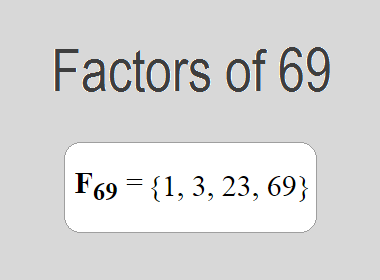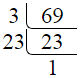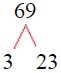# Factors of 69The factors of 69 are 1, 3, 23, and 69 i.e. F69 = {1, 3, 23, 69}. The factors of 69 are all the numbers that can divide 69 without leaving a remainder.

We can check if these numbers are factors of 69 by dividing 69 by each of them. If the result is a whole number, then the number is a factor of 69. Let's do this for each of the numbers listed above:

·        1 is a factor of 69 because 69 divided by 1 is 69.

·        3 is a factor of 69 because 69 divided by 3 is 23.

·        23 is a factor of 69 because 69 divided by 23 is 3.

·        69 is a factor of 69 because 69 divided by 69 is 1.

## How to Find Factors of 69?

1 and the number itself are the factors of every number. So, 1 and 69 are two factors of 69. To find the other factors of 69, we can start by dividing 69 by the numbers between 1 and 69. If we divide 69 by 2, we get a remainder of 1. Therefore, 2 is not a factor of 69. If we divide 69 by 3, we get a remainder of 0. Therefore, 3 is a factor of 69.

Next, we can check if 4 is a factor of 69. If we divide 69 by 4, we get a remainder of 1. Therefore, 4 is not a factor of 69. We can continue this process for all the possible factors of 69.

Through this process, we can find that the factors of 69 are 1, 3, 23, and 69. These are the only numbers that can divide 69 without leaving a remainder.

********************

********************

## Properties of the Factors of 69

The factors of 69 have some interesting properties. One of the properties is that the sum of the factors of 69 is equal to 96. We can see this by adding all the factors of 69 together:

1 + 3 + 23 + 69 = 96

Another property of the factors of 69 is that they are all odd numbers. This is because 69 is an odd number, and any even number cannot divide an odd number without leaving a remainder.

Another property of the factors of 69 is that the prime factors of 69 are 3 and 23 only.

## Applications of the Factors of 69

The factors of 69 have several applications in mathematics. One of the applications is in finding the highest common factor (HCF) of two or more numbers. The HCF is the largest factor that two or more numbers have in common. For example, to find the HCF of 69 and 46, we need to find the factors of both numbers and identify the largest factor they have in common. The factors of 69 are 1, 3, 23, and 69. The factors of 46 are 1, 2, 23, and 46. The largest factor that they have in common is 23. Therefore, the HCF of 69 and 46 is 23.

Another application of the factors of 69 is in prime factorization. Prime factorization is the process of expressing a number as the product of its prime factors. The prime factors of 69 are 3 and 23, since these are the only prime numbers that can divide 69 without leaving a remainder. Therefore, we can express 69 as:

69 = 3 × 23

We can do prime factorization by division and factor tree method also. Here is the prime factorization of 69 by division method,69 = 3 × 23

Here is the prime factorization of 69 by the factor tree method,69 = 3 × 23

## Conclusion

The factors of 69 are the numbers that can divide 69 without leaving a remainder. The factors of 69 are 1, 3, 23, and 69. The factors of 69 have some interesting properties, such as being odd numbers and having a sum of 96. The factors of 69 have several applications in mathematics, such as finding the highest common factor and prime factorization.